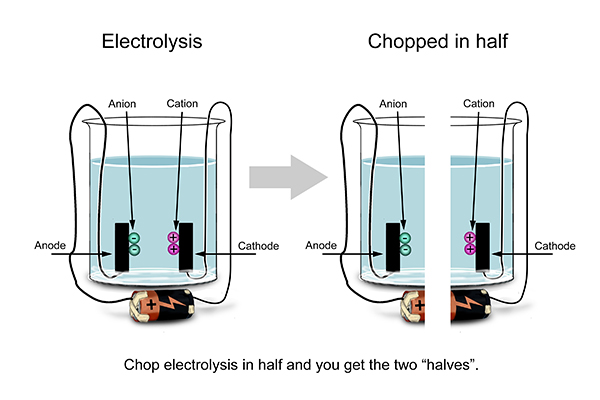# Half equations

The reactions that take place at each electrode are called half equations.Look at what happens at one electrode and then look at what happens at the other.

Write out one, then the other.

### Example 1

The following is an example of the electrolysis of molten sodium chloride (NaCl) and the half equations that occur (note: there is no water).

At the anode:                2Cl-                              →       Cl2               +          2e- (where e- represents an electron)

At the cathode:             2Na+         +          2e-    →       2Na

In other words, the sodium chloride has been split into pure chlorine (at the anode) and pure sodium (at the cathode).

### Example 2

The electrolysis of molten lead bromide, PbBr2 (note: there is no water).

At the anode:                2Br-                              →        Br2              +          2e-

At the cathode:              Pb2+           +       2e-      →        Pb

### Example 3

Electrolysis of bauxite to make aluminium (Al).

At the anode:                4Al3+      +      12e-         →        4Al

At the cathode:              6O2-       -       12e-         →        3O2